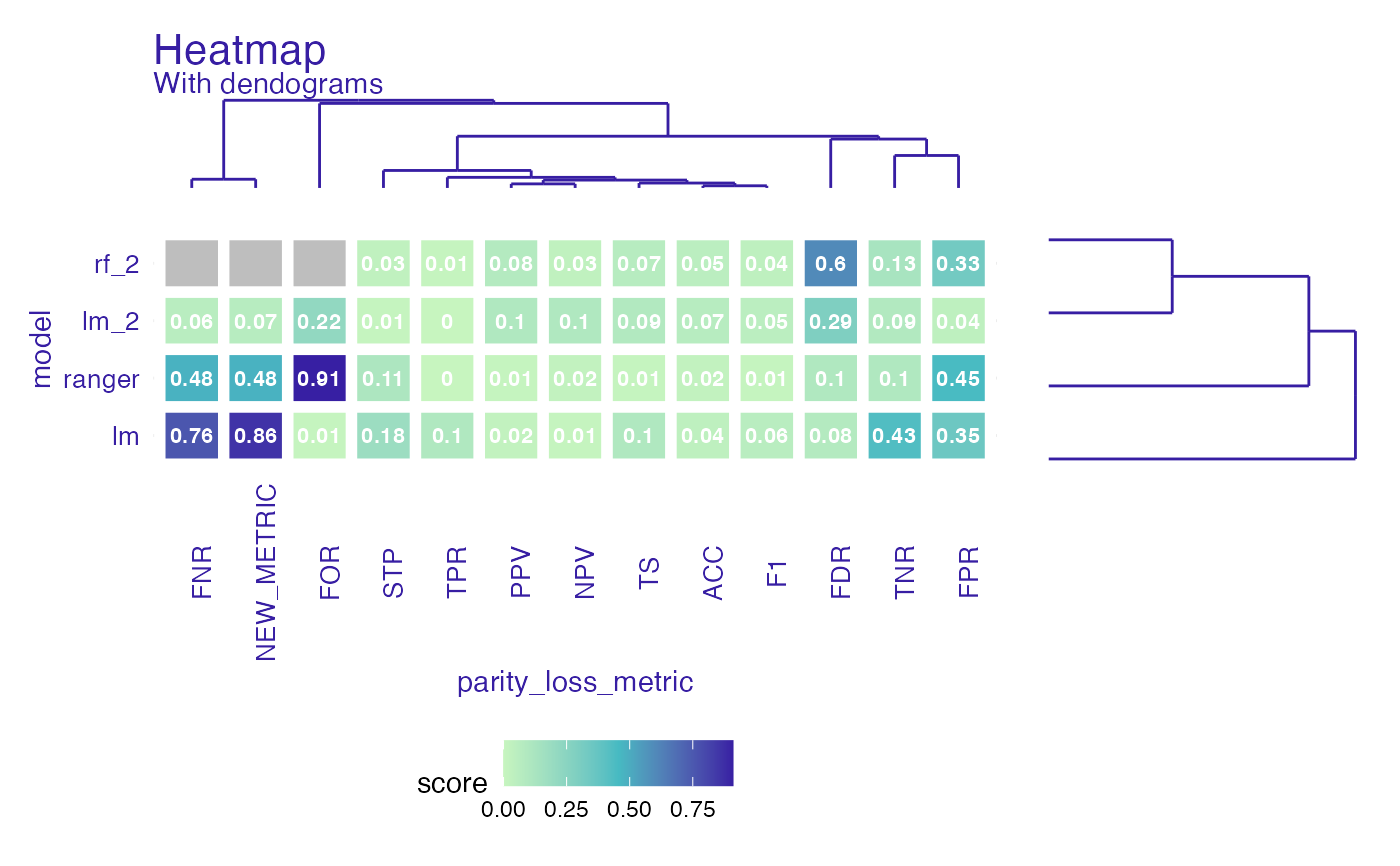Create fairness_heatmap object to compare both models and metrics. If parameter scale is set to TRUE metrics will be scaled to median = 0 and sd = 1. If NA's appear heatmap will still plot, but with gray area where NA's were.

fairness_heatmap(x, scale = FALSE)

Arguments

x object of class fairness_object logical, if codeTRUE metrics will be scaled to mean 0 and sd 1. Default FALSE

Value

fairness_heatmap object.

It is a list with following fields:

• heatmap_data - data.frame with information about score for model and parity loss metric

• matrix_model - matrix used in dendogram plots

• scale - logical parameter passed to fairness_heatmap

• label - character, vector of model labels

Examples

data("german")

y_numeric <- as.numeric(german$Risk) - 1 lm_model <- glm(Risk ~ ., data = german, family = binomial(link = "logit") ) rf_model <- ranger::ranger(Risk ~ ., data = german, probability = TRUE, num.trees = 200, num.threads = 1 ) explainer_lm <- DALEX::explain(lm_model, data = german[, -1], y = y_numeric) #> Preparation of a new explainer is initiated #> -> model label : lm ( default ) #> -> data : 1000 rows 9 cols #> -> target variable : 1000 values #> -> predict function : yhat.glm will be used ( default ) #> -> predicted values : No value for predict function target column. ( default ) #> -> model_info : package stats , ver. 4.1.1 , task classification ( default ) #> -> predicted values : numerical, min = 0.1369187 , mean = 0.7 , max = 0.9832426 #> -> residual function : difference between y and yhat ( default ) #> -> residuals : numerical, min = -0.9572803 , mean = 1.940006e-17 , max = 0.8283475 #> A new explainer has been created! explainer_rf <- DALEX::explain(rf_model, data = german[, -1], y = y_numeric) #> Preparation of a new explainer is initiated #> -> model label : ranger ( default ) #> -> data : 1000 rows 9 cols #> -> target variable : 1000 values #> -> predict function : yhat.ranger will be used ( default ) #> -> predicted values : No value for predict function target column. ( default ) #> -> model_info : package ranger , ver. 0.13.1 , task classification ( default ) #> -> predicted values : numerical, min = 0.05022024 , mean = 0.6969863 , max = 0.9981746 #> -> residual function : difference between y and yhat ( default ) #> -> residuals : numerical, min = -0.7025734 , mean = 0.003013703 , max = 0.6601389 #> A new explainer has been created! fobject <- fairness_check(explainer_lm, explainer_rf, protected = german$Sex,
privileged = "male"
)
#> Creating fairness classification object
#> -> Privileged subgroup		: character ( Ok  )
#> -> Protected variable		: factor ( Ok  )
#> -> Cutoff values for explainers	: 0.5 ( for all subgroups )
#> -> Fairness objects		: 0 objects
#> -> Checking explainers		: 2 in total (  compatible  )
#> -> Metric calculation		: 13/13 metrics calculated for all models
#>  Fairness object created succesfully

# same explainers with different cutoffs for female
fobject <- fairness_check(explainer_lm, explainer_rf, fobject,
protected = german\$Sex,
privileged = "male",
cutoff = list(female = 0.4),
label = c("lm_2", "rf_2")
)
#> Creating fairness classification object
#> -> Privileged subgroup		: character ( Ok  )
#> -> Protected variable		: factor ( Ok  )
#> -> Cutoff values for explainers	: female: 0.4, male: 0.5
#> -> Fairness objects		: 1 object (  compatible  )
#> -> Checking explainers		: 4 in total (  compatible  )
#> -> Metric calculation		: 10/13 metrics calculated for all models ( 3 NA created )
#>  Fairness object created succesfully

fh <- fairness_heatmap(fobject)

plot(fh)
#> Warning: Removed 3 rows containing missing values (geom_text).